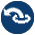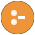#random.pl -- Random numbers

This library is derived from the DEC10 library random. Later, the core random generator was moved to C. The current version uses the SWI-Prolog arithmetic functions to realise this library. These functions are based on the GMP library.

author
- R.A. O'Keefe, V.S. Costa, L. Damas, Jan Wielemaker
- Built-in function random/1: A is `random(10)`
random(-R:float) is det
Binds R to a new random float in the open interval (0.0,1.0).
- setrand/1, getrand/1 may be used to fetch/set the state.
- In SWI-Prolog, random/1 is implemented by the function random_float/0.
random_between(+L:int, +U:int, -R:int) is semidet
Binds R to a random integer in [L,U] (i.e., including both L and U). Fails silently if U<L.
random(+L:int, +U:int, -R:int) is det
random(+L:float, +U:float, -R:float) is det
Generate a random integer or float in a range. If L and U are both integers, R is a random integer in the half open interval [L,U). If L and U are both floats, R is a float in the open interval (L,U).
deprecated
- Please use random/1 for generating a random float and random_between/3 for generating a random integer. Note that random_between/3 includes the upper bound, while this predicate excludes it.
setrand(+State) is det
getrand(-State) is det
Query/set the state of the random generator. This is intended for restarting the generator at a known state only. The predicate setrand/1 accepts an opaque term returned by getrand/1. This term may be asserted, written and read. The application may not make other assumptions about this term.

For compatibility reasons with older versions of this library, setrand/1 also accepts a term `rand(A,B,C)`, where A, B and C are integers in the range 1..30,000. This argument is used to seed the random generator. Deprecated.

Errors
- `existence_error(random_state, _)` is raised if the underlying infrastructure cannot fetch the random state. This is currently the case if SWI-Prolog is not compiled with the GMP library.
- set_random/1 and random_property/1 provide the SWI-Prolog native implementation.
maybe is semidet
Succeed/fail with equal probability (variant of maybe/1).
maybe(+P) is semidet
Succeed with probability P, fail with probability 1-P
maybe(+K, +N) is semidet
Succeed with probability K/N (variant of maybe/1)
random_perm2(?A, ?B, ?X, ?Y) is semidet
Does X=A,Y=B or X=B,Y=A with equal probability.
random_member(-X, +List:list) is semidet
X is a random member of List. Equivalent to random_between(1, |List|), followed by nth1/3. Fails of List is the empty list.
Compatibility
- Quintus and SICStus libraries.
random_select(-X, +List, -Rest) is semidet
random_select(+X, -List, +Rest) is det
Randomly select or insert an element. Either List or Rest must be a list. Fails if List is the empty list.
Compatibility
- Quintus and SICStus libraries.
randset(+K:int, +N:int, -S:list(int)) is det
S is a sorted list of K unique random integers in the range 1..N. The implementation uses different techniques depending on the ratio K/N. For small K/N it generates a set of K random numbers, removes the duplicates and adds more numbers until |S| is K. For a large K/N it enumerates 1..N and decides randomly to include the number or not. For example:
```?- randset(5, 5, S).
S = [1, 2, 3, 4, 5].          (always)
?- randset(5, 20, S).
S = [2, 7, 10, 19, 20].```
- randseq/3.
randseq(+K:int, +N:int, -List:list(int)) is det
S is a list of K unique random integers in the range 1..N. The order is random. Defined as
```randseq(K, N, List) :-
randset(K, N, Set),
random_permutation(Set, List).```
- instantiation_error, `type_error(list, _)`.